# Railway

Railway line had on 5.8 km segment climb 9 permille. How many meters track ascent?

x =  52.1979 m

### Step-by-step explanation: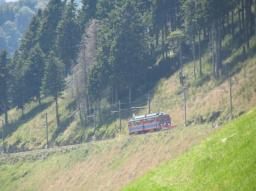Did you find an error or inaccuracy? Feel free to write us. Thank you!Tips to related online calculators

#### You need to know the following knowledge to solve this word math problem:

We encourage you to watch this tutorial video on this math problem:

## Related math problems and questions:

• Mountain railway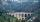Height difference between points A, B of railway line is 38.5 meters, their horizontal distance is 3.5 km. Determine average climb in permille up the track.Between cities A and B is route 13 km long of stúpanie average 7‰. Calculate the height difference of cities A and B.
• Railway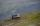Between points A, B, whose horizontal distance is 1.5 km railway line has 8promile climb. Between points B, C with horizontal distance of 900 m is climb 14promile. Calculate differences of altitudes between points A and C.
• Slope of trackCalculate the average slope (in permille and even in degrees) of the rail tracks between Prievidza (309 m AMSL) and Nitrianske Pravno (354 m AMSL), if the track is 11 km long.
• Height differenceWhat height difference is overcome if we pass a road 1 km long with a pitch21 per mille?
• RailwaysRailways climb 7.4 ‰. Calculate the height difference between two points on the railway distant 3539 meters.
• ClimbOn the road sign, which informs the climb is 8.7%. The car drive 5 km along this road. What is the height difference that the car went to?
• ClimbFor horizontal distance 4.2 km road rise by 6.3 m. Calculate the road pitch in ‰ (permille, parts per thousand).The average climb of the road is given by ratio 1:15. By what angle road average climb?
• Cablecar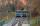Funicular on Petrin (Prague) was 408 meters long and overcomes the difference 106 meters in altitude. Calculate the angle of climb.
• ClimbRoad has climbing 1:27. How big is a angle corresponds to this climbing?
• Map - climb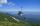On the map of High Tatras in scale 1:11000 are cable car stations in the Tatranska Lomnica and in the Skalnate Pleso with distance 354.6 mm. Altitude of this stations are 949 m and 1760 m. What is average angle of climb of this cable car track?
• LiftThe largest angle at which the lift rises is 16°31'. Give climb angle in permille.
• AngleA straight line p given by the equation y = (-8)/(6) x +78. Calculate the size of angle in degrees between line p and y-axis.
• The railway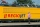The railway line has a gradient of 12 per mille. How many meters will it ascend to a horizontal distance of 4 km?
• Power line poleFrom point A, the power line pole is seen at an angle of 18 degrees. From point B to which we get when going from point A 30m away from the column at an angle of 10 degrees. Find the height of the power pole.
• Three pillars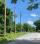On a straight road, three pillars are 6 m high at the same distance of 10 m. At what angle of view does Vlado see each pillar if it is 30 m from the first and his eyes are at 1.8 m high?